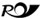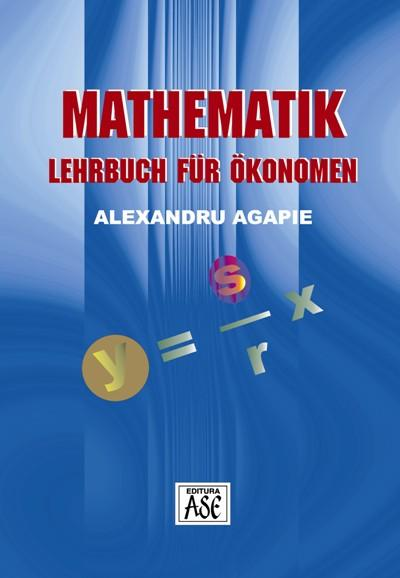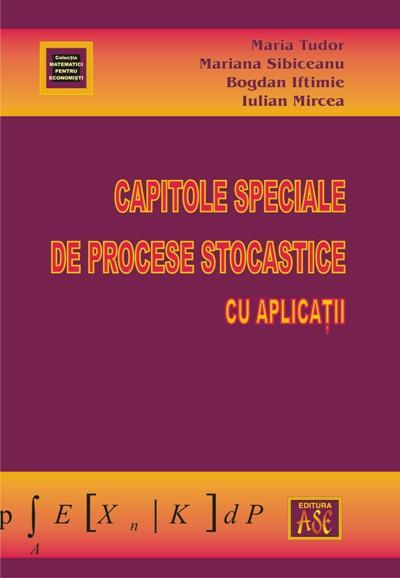# Fundamentals of applied mathematics in economics

Autors: Gabriela Beganu, Marius Giuclea

Year of appearance: 2011

ISBN: 978-606-505-398-4

20,70 lei In stock: YESRomanian Post delivery

Teaching the fundamentals of the theory of probability and mathematical statistics is done in all economic faculties, knowing that the phenomena and processes studied economics in probabilistic nature, and making smart decisions in this area, where there are hazardous conditions and uncertainty can not be done without thorough probabilistic knowledge.

The book is structured as syllabus economic faculties, being useful not only for students from the Bucharest Academy of Economic Studies, but to all students of economic faculties.
In the first part of the manual, which contains two chapters, are exposed the knowledge of purely mathematical analyse necessary to understand the reasoning used in various demonstrations of the following two parts. In the second part,  containing three chapters, are presented basic concepts of probability theory. The next three chapters of Part Three shows the techniques of mathematical statistics to choose, present and analyze data refering the phenomena that occur in conditions of uncertainties.

The manual completes theoretical knowledge with examples appropriate and offers practical application for  illustrate theoretical concepts.

## Similar products### Fundamental methods of mathematics with applications in economics

17,30 lei### Mathematics. Textbooks for economics

19,00 lei### Exercises on computational techniques: an introduction

40,40 lei31,80 lei

## Others have bought17,30 lei